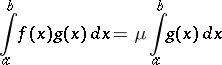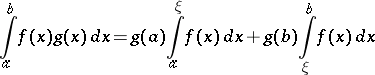# Difference between revisions of "Riemann integral"

A generalization of the concept of a Cauchy integral to a certain class of discontinuous functions; introduced by B. Riemann (1853). Consider a function $f$ which is given on an interval $[a,b]$. Let $a=x_0<x_1<\dots<x_n=b$ is a partition (subdivision) of the interval $[a,b]$ and $\Delta x_i = x_i-x_{i-1}$, where $i=1,\dots,n$. The sum \begin{equation}\label{eq:1} \sigma = f(\xi_1)\Delta x_1+\dots+f(\xi x_i)\Delta x_i +\dots +f(x_n)\Delta x_n, \end{equation} where $x_{i-1}\leq\xi_i\leq x_i$, is called the Riemann sum corresponding to the given partition of $[a,b]$ by the points $x_i$ and to the sample of points $\xi_i$. The number $I$ is called the limit of the Riemann sums \eqref{eq:1} as $\max_i \Delta x_i \to 0$ if for any $\varepsilon>0$ a $\delta>0$ can be found such that $\max_i \Delta x_i < \delta$ implies the inequality $|\sigma - I|<\varepsilon$. If the Riemann sums have a finite limit $I$ as $\max_i \Delta x_i \to 0$, then the function $f$ is called Riemann integrable over $[a,b]$, where $a< b$. The limit is known as the definite Riemann integral of $f$ over $[a,b]$, and is written as \begin{equation}\label{eq:2} \int\limits_a^bf(x)\,dx. \end{equation} When $a=b$ then, by definition, \begin{equation} \int\limits_a^af(x)\,dx = 0, \end{equation} and when $a>b$ the integral \eqref{eq:2} is defined using the equation \begin{equation} \int\limits_a^bf(x)\,dx = -\int\limits_b^af(x)\,dx. \end{equation} A necessary and sufficient condition for the Riemann integrability of $f$ over $[a,b]$ is the boundedness of $f$ on this interval and the zero value of the Lebesgue measure of the set of all points of discontinuity of $f$ contained in $[a,b]$.

## Properties of the Riemann integral.

1. Every Riemann-integrable function $f$ on $[a,b]$ is also bounded on this interval (the converse is not true: The Dirichlet function is an example of a bounded and non-integrable function on $[a,b]$).
2. The linearity property: For any constants $\alpha$ and $\beta$, the integrability over $[a,b]$ of both functions $f$ and $g$ implies that the function $\alpha f + \beta g$ is integrable over this interval, and the equation \begin{equation} \int\limits_a^b[\alpha f(x) + \beta g(x)]\,dx = \alpha\int\limits_a^bf(x)\,dx + \beta\int\limits_a^bg(x)\,dx \end{equation} holds.
3. The integrability over $[a,b]$ of both functions $f$ and $g$ implies that their product $fg$ is integrable over this interval.
4. Additivity: The integrability of a function $f$ over both intervals $[a,c]$ and $[c,b]$ implies that $f$ is integrable over $[a,b]$, and \begin{equation} \int\limits_a^bf(x)\,dx = \int\limits_a^cf(x)\,dx + \int\limits_c^bf(x)\,dx. \end{equation}
5. If two functions $f$ and $g$ are integrable over $[a,b]$ and if $f(x)\geqslant g(x)$ for every $x$ in this interval, then \begin{equation} \int\limits_a^bf(x)\,dx \geqslant \int\limits_a^bg(x)\,dx. \end{equation}
6. The integrability of a function $f$ over $[a,b]$ implies that the function $|f|$ is integrable over this interval, and the estimate \begin{equation} \left|\int\limits_a^bf(x)\,dx\right| \leqslant \int\limits_a^b|f(x)|\,dx \end{equation} holds.
7. The mean-value formula: If two real-valued functions $f$ and $g$ are integrable over $[a,b]$, if the function $g$is non-negative or non-positive everywhere on this interval, and if $M$ and $m$ are the least upper and greatest lower bounds of $f$ on $[a,b]$, then a number $\mu$ can be found, $m\leqslant\mu\leqslant M$, such that the formula(3)

holds. If, in addition,is continuous on, then this interval will contain a pointsuch that in formula (3),8) The second mean-value formula (Bonnet's formula): If a functionis real-valued and integrable overand if a functionis real-valued and monotone on this interval, then a pointcan be found insuch that the formulaholds.

How to Cite This Entry:
Riemann integral. Encyclopedia of Mathematics. URL: http://encyclopediaofmath.org/index.php?title=Riemann_integral&oldid=29274
This article was adapted from an original article by V.A. Il'in (originator), which appeared in Encyclopedia of Mathematics - ISBN 1402006098. See original article